Skip to main content

# Upper bound limit analysis of plates using a rotation-free isogeometric approach

## Abstract

### Background

This paper presents a simple and effective formulation based on a rotation-free isogeometric approach for the assessment of collapse limit loads of plastic thin plates in bending.

### Methods

The formulation relies on the kinematic (or upper bound) theorem and namely B-splines or non-uniform rational B-splines (NURBS), resulting in both exactly geometric representation and high-order approximations. Only one deflection variable (without rotational degrees of freedom) is used for each control point. This allows us to design the resulting optimization problem with a minimum size that is very useful to solve large-scale plate problems. The optimization formulation of limit analysis is transformed into the form of a second-order cone programming problem so that it can be solved using highly efficient interior-point solvers.

### Results and conclusions

Several numerical examples are given to demonstrate reliability and effectiveness of the present method in comparison with other published methods.

## 1Background

Accurate prediction of the load bearing capacity of plate structures plays an important role in many practical engineering problems. Traditional elastic designs cannot evaluate the actual load carrying capacity of plates and incremental elasto-plastic analyses can become cumbersome and present convergence issues for large-scale structures. Therefore, various limit analysis approaches have been devised to investigate the behavior of structures in the plastic region. Nowadays, limit analysis has become a well-known tool for assessing the safety load factor of engineering structures as an efficient direct method. Due to limitation of analytical methods, various numerical approaches such as finite element methods (FEM) –, meshfree methods ,, and natural element method , just to mention a few, have therefore been developed.

It is also worth adding that mathematical programming is the other key issue in numerical assessment of limit analysis problem. Discrete upper bound limit analysis results in a minimization problem involving linear or nonlinear programming. Linear programming problems can be applied for piecewise linearization of yield criteria, but an important number of additional variables is often needed. However, most of the yield criteria for plates can be formed as an intersection of cones for which the limit analysis problem can be solved efficiently by the primal-dual interior point method , implemented in the MOSEK software package . This algorithm was proved to be a very effective optimization tool for the limit analysis of structures ,,,–, and therefore it will be used in our study.

Isogeometric approach (IGA) has been recently proposed by Hughes et al.  to unify the fields of Computer Aided Design (CAD) and Finite Element Analysis (FEA). The basic idea is that the IGA uses the same basis functions, namely B-splines or non-uniform rational B-splines (NURBS), to describe precisely the geometry, especially containing conic sections and to construct the finite approximation for analysis. It is well known that NURBS functions provide a flexible way to make refinement, de-refinement and degree elevation . They enable to easily achieve continuity up to C(p−1), instead of C0-continuity as it typically happens with traditional FEM. Hence, IGA naturally verifies the C1-continuity of thin plates, which is interested in this study. The IGA has been well known and widely applied to various practical problems – and so on.

Among various plate theories , the classical plate theory (CPT) and the first-order shear deformation plate theory (FSDT) have been widely used in many numerical methods, especially finite elements. The first-order shear deformation plate theory assumes that transverse shear stresses are constants through the thickness and a shear correction factor (SCF) is needed to take into account the non-linear distribution of shear stresses. It is known in FSDT models that the FE approximation functions only require C0-continuity across element boundaries. Such a construction is simple but leads to shear locking problems. In CPT, C1-continuity of approximation fields across element boundaries is needed. Unfortunately, it is difficult to construct FE formulations with C1-continuous approximation. Traditionally, the conforming FE approximation of the Kirchhoff plate model has in general 3 degrees of freedom per node. This is due to the continuity of the rotation solutions. It is also well known in the literature that non-conforming finite element models enable us to relax strict requirements of the continuity of the rotations. Attempts to eliminate the rotational degrees of freedom help us to reduce significantly the total number of degrees of freedom of problem without loss of accuracy of solution. As a result, such approaches promise more benefit for solving large-scale industrial problems ,,. For example, an efficient way of the rotation-free FE approaches for plate and shell analysis is to use C0 basis functions via the so-called cell-centred and cell-vertex finite volume schemes –. The rotation-free isogeometric approach recently proposed is regarded as an alternative way for solving practical problems. The method is conformable to the thin plate/shell theory and the C1-continuity is easily achieved using NURBS basis functions . Several investigations on the rotation-free formulation can be found in the literature, e.g., Bernoulli-Euler beams , Poisson-Kirchhoff plates , multi-patch Kirchhoff-Love shells  and large deformation analysis with rotation-free . It was demonstrated in the aforementioned references that the rotation-free isogeometric approach is a potential candidate for solving a wide range of practical problems. It therefore deserves for pursuing and developing this approach for limit analysis of thin plate structures.

This paper further exploits the advantage of a rotation-free isogeometric approach to the assessment of collapse limit loads of plastic thin plates in bending. The kinematic formulation relies on the displacement (deflection) approximation using NURBS, resulting in both exact geometric representation and high-order approximations. Only deflection degrees of freedom are involved in the underlying optimization problem. This enables us to design the resulting optimization problem with a minimum size and to reduce computational cost. We adopt a simple procedure to eliminate rotational degrees of freedom on essential boundary conditions related to the constraint of normal slopes. The resulting non-smooth optimization problem is then written in the form of minimizing a sum of Euclidean norms so that it can be solved using highly efficient interior-point solvers. Several numerical examples are provided to show the reliability and accuracy of the present formulation.

The paper is arranged as follows: a brief review of B-spline and NURBS surfaces is described in the next section. This is followed by a section stating a rotation-free NURBS-based isogeometric formulation for limit analysis of thin plate problems. The solution procedure is given in the fourth section. Several numerical examples are illustrated in the fifth section. Finally, we close our paper with some concluding remarks.

## 2Methods

### 2.1 A brief review of NURBS basis functions and surfaces

#### 2.1.1 Knot vectors and basis functions and surfaces

Let Ξ=[ξ1,ξ2,…,ξn+p+1] be a nondecreasing sequence of parameter values, ξ i ξi+1,i=1,…,n+p, where p is the polynomial order and n is the number of basis functions. The ξ i are called knots, and Ξ is the set of coordinates in the parametric space. The knot vector is called uniform if all knots are equally spaced. If the first and the last knots are reduplicated p+1 times, the knot vector is known as open. A B-spline basis function is C continuous inside a knot span and Cp−1 continuous at a single knot. A knot value can appear more than once and is then called a multiple knot. At a knot of multiplicity k, the continuity is Cpk. Given a knot vector, the B-spline basis functions Ni,p(ξ) of order p = 0 are defined as follows:

$N i , 0 (ξ)= 1 ξ i ≤ ξ < ξ i + 1 0 otherwise$
(1)

The basis function of order p>0 is defined by the following recursion formula 

$N i , p ξ = ξ − ξ i ξ i + p − ξ i N i , p − 1 ξ + ξ i + p + 1 − ξ ξ i + p + 1 − ξ i + 1 N i + 1 , p − 1 ξ withp=(1,2,3,…)$
(2)

For p = 0 and 1, the basis functions of isogeometric analysis are identical to those of standard piecewise constant and linear finite elements, respectively. Nevertheless, for p≥2, they are different . Therefore, the present work will consider only basis functions with p≥2. Figures 1 and 2 illustrate a set of one-dimensional (1D) and two-dimensional (2D) quadratic and cubic B-spline basis functions for open uniform knot vectors $Ξ= 0 , 0 , 0 , 1 2 , 1 , 1 , 1$ and $Ξ= 0 , 0 , 0 , 0 , 1 2 , 1 , 1 , 1 , 1$, respectively.Figure 1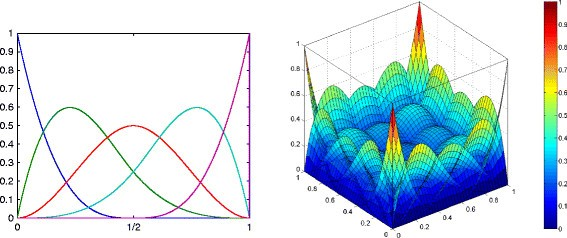Figure 2

#### 2.1.2 NURBS surfaces

A B-spline curve is defined as

$C ξ = ∑ i = 1 n N i , p ξ P i$
(3)

where P i are the control points, n denotes the number of control points and Ni,p(ξ) is the p th-degree B-spline basis function defined on the open knot vector.

Given two knot vectors Ξ={ξ1,ξ2,…,ξn+p+1} and and a control net pi,j, a tensor-product B-spline surface is defined as

$S ξ , η = ∑ i = 1 n ∑ j = 1 m N i , p ξ M j , q η p i , j$
(4)

where Ni,p(ξ) and Mj,q(η) are the B-spline basis functions defined on the knot vectors Ξ and , respectively.

In a finite element context, we identify the logical coordinates (i, j) of B-spline surfaces with the traditional notation of a ‘node’ I and rewrite Equation 4 as follows:

$S ξ , η = ∑ I n × m N I b ξ , η P I$
(5)

where $N I b ξ , η = N i , p ξ M j , q η$ is the shape function associated with a node I. The superscript b indicates that $N I b (ξ,η)$ is a B-spline shape function.

Non-uniform rational B-splines (NURBS) are obtained by augmenting every point in the control mesh P I with the control weight ζ I . The weighting function is constructed as follows:

$ζ g ξ , η = ∑ I = 1 n × m N I b ξ , η ζ I$
(6)

The NURBS surfaces are then defined by

$S ξ , η = ∑ I = 1 n × m N I b ξ , η ζ I P I ζ g ξ , η = ∑ I = 1 n × m N I ξ , η P I$
(7)

where $N I ξ , η = N I b ξ , η ζ I / ζ g ξ , η$ are NURBS basis functions. An example of a quadratic NURBS surface with 4×4 elements is indicated in Figure 3.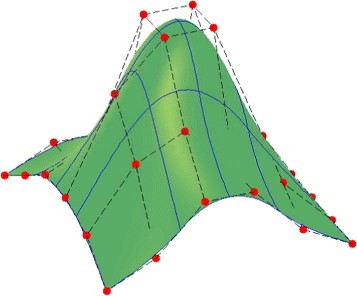Figure 3

### 2.2 Rotation-free isogeometric formulation for upper bound limit analysis of plates

#### 2.2.1 A background on limit analysis theorems of thin plates

Let $Ω⊂ ℝ 2$ be the mid-plane of a plate and $w ̇$ be the transversal displacement velocity (or deflection velocity) in the z direction. Further, let us consider a kinematical boundary $Γ 1 = Γ w ∪ Γ w n$ and a static boundary $Γ 2 = Γ m ∪ Γ m n$, where the subscript n stands for the outward normal vector. The general relations for thin Kirchhoff plates are described as follows.

Equilibrium

Collecting the bending moments in the vector mT= [m xx ,m yy ,m xy ], the equilibrium equation can be written as

$( ∇ 2 ) T m+λ q ̄ =0$
(8)

where $q ̄$ is the transverse load, λ is the collapse load multiplier and the differential operator 2 is defined by $∇ 2 = ∂ 2 ∂ x 2 ∂ 2 ∂ y 2 2 ∂ 2 ∂x∂y T$.

Compatibility

If w denotes the transverse displacement velocity, the curvature rates can be expressed by the following relation

$κ ̇ = κ ̇ xx , κ ̇ yy , 2 κ ̇ xy T = ∇ 2 w ̇$
(9)

Flow rule and yield condition

In the framework of a limit analysis problem, only plastic strains are considered and are assumed to obey the normality rule $κ ̇ = μ ̇ ∂ψ ∂ m$, where the plastic multiplier $μ ̇$ is non-negative and the yield function ψ(m) is convex. In this study, the von Mises failure criterion in the space of moment components is used

$ψ(m)= m T P m − m p ≤0$
(10)

where m p =σ0t2/4 is the plastic moment of resistance per unit width of a plate of uniform thickness t, σ0 is the yield stress and

$P= 1 2 2 − 1 0 − 1 2 0 0 0 6$
(11)

The dissipation rate

The internal dissipation power of the two-dimensional plate domain can be written as a function of the curvature rates as

$D p ( κ ̇ )= ∫ Ω ∫ − t / 2 t / 2 σ 0 ε ̇ T Θ ε ̇ dzdA= m p ∫ Ω κ ̇ T Θ κ ̇ dΩ$
(12)

where

$ε ̇ = ε ̇ xx ε ̇ yy γ ̇ xy =z κ ̇$
(13)

and

$Θ= P − 1 = 1 3 4 2 0 2 4 0 0 0 1$
(14)

Details on the derivation of the dissipation for plate problems can be found in . Let it be pointed out that, here, the velocity fields are supposed to be C1-continuous. In fact, more general fields presenting discontinuities of the normal rotation are possible. In this case, the expression of the dissipation power includes a supplementary term. For more details on this aspect, we refer to .

Let denote a space of kinematically admissible velocity field: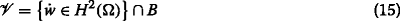and Σ be an appropriate space of symmetric stress tensors and B is a set of essential boundary conditions defined in subsection ‘Essential boundary conditions’. More details on the mathematical formulations for limit analysis can be found in . The external work rate of a transversal force $q ̄$ associated with a virtual plastic flow is expressed in the linear form as

$W ex ( w ̇ )= ∫ Ω q ̄ w ̇ dΩ$
(16)

The internal work rate for sufficiently smooth stresses (or moments) m and velocity field $w ̇$ is given by the bilinear form

$W in (m, w ̇ )= ∫ Ω m T κ( w ̇ )dΩ$
(17)

The equilibrium equation is then described in the form of virtual work rate as follows: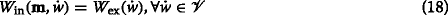Furthermore, the stresses m must satisfy the yield condition for the assumed material. This stress field belongs to a convex set, , obtained from the used field condition. For the von Mises criterion, one writes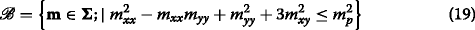If defining , the exact collapse multiplier λexact can be determined by solving any of the following optimization problems :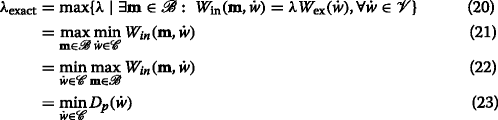Problems (20) and (23) are known as static and kinematic principles of limit analysis, respectively. The limit load of both approaches converges to the exact solution. Herein, a saddle point ($m ∗ , w ̇ ∗$) exists such that both the maximum of all lower bounds λ and the minimum of all upper bounds λ+ coincide and are equal to the exact value λexact. In this work, we only focus on the kinematic formulation. Hence, problem (23) will be used to evaluate an upper-bound limit load factor using a NURBS-based isogeometric approach.

#### 2.2.2 NURBS-based approximate formulation

Using the same NURBS basis functions, both the description of the geometry (or the physical point) and the velocity of the displacement field are expressed as

$x(ξ,η)= ∑ I n × m N I ξ , η P I , w ̇ h (x(ξ,η))= ∑ I n × m N I ξ , η w ̇ I$
(24)

where n×m represent the number of basis functions, xT=(x,y) is the physical coordinates vector, N I (ξ,η) is the NURBS basis function and $w ̇ I$ is the nodal value of $w ̇ h$ at the control point I, respectively.

The curvature rates are written as

$κ ̇ = ∑ I B I w ̇ I$
(25)

where

$B I = N I , xx N I , yy 2 N I , xy T$
(26)

The plastic dissipation power (Equation 12) of the perfectly rigid plastic body is computed over all patches:

$D p h = m p ∫ Ω κ ̇ T Θ κ ̇ dΩ= m p ∑ e = 1 nel ∫ Ω e κ ̇ T Θ κ ̇ dΩ$
(27)

where nel denotes the total number of elements. The integration $∫ Ω e κ ̇ T Θ κ ̇ dΩ$ in Equation 27 is approximated using the Gaussian quadrature rule  which allows Equation 27 to be rewritten as

$D p h ≈ m p ∑ i = 1 NG ω ̄ i J i κ ̇ i T Θ κ ̇ i$
(28)

where N G=n e l×n G is the total number of Gauss points of the problem, nG is the number of Gauss points in each element, $ω ̄ i$ is the weight value at the Gauss point i and |J i | is the determinant of the Jacobian matrix computed at the Gauss point i.

The curvature rate $κ ̇$ is now evaluated at Gauss points as

$κ ̇ i = B i w ̇ ∀i= 1 , NG ¯$
(29)

where B i is defined as the global deformation matrix and $w ̇ T =[ w ̇ 1 , w ̇ 2 ,…, w ̇ nCP ]$ is the global displacement velocity vector, in which nCP is the total control points of the problem. The external work rate given in Equation 16 is also computed at Gauss points as

$W ext ( w ̇ )= ∑ i = 1 NG q ̄ ω i ̄ J i N( ξ ̄ i , η ̄ i ) w ̇ =1$
(30)

where $N=[ N 1 ( ξ ̄ i , η ̄ i ), N 2 ( ξ ̄ i , η ̄ i ),…, N nCP ( ξ ̄ i , η ̄ i )]$ is the global basic function vector and ($ξ ̄ i , η ̄ i$) is the Gaussian quadrature point in a bi-unit parent element.

Finally, the optimization problem (23) associated with the IGA can now be rewritten as

$λ + = min m p ∑ i = 1 NG ω ̄ i J i κ ̇ i T Θ κ ̇ i s.t κ ̇ i = B i w ̇ , ∀ i = 1 , NG ¯ w ̇ = 0 and w n ̇ = 0 on Γ 1 W ext ( w ̇ ) = ∑ i = 1 NG q ̄ ω i J i N ( ξ ̄ i , η ̄ i ) w ̇ = 1$
(31)

Since integral Equation 27 is not calculated exactly, it cannot be said that the formulation yields a strict upper bound using this formula. Nevertheless, the optimal velocity field is still kinematically admissible and corresponding compatible strain are obtained using the B i matrix so that a strict upper bound can be obtained provided that the dissipation is computed exactly a posteriori. However, in practice, there is practically no difference and the computed values can be considered as upper bounds.

#### 2.2.3 Essential boundary conditions

In this part, we show how to impose essential boundary conditions of the isogeometric approach. For the sake of simplicity, we consider the following several Dirichlet boundary conditions (BCs):

Simply supported plates with curved boundaries

$B={ w ̇ ( x D )=0on Γ 1 }$
(32)

Clamped plates

$B={ w ̇ ( x D )=0and w ̇ n ( x D )=0on Γ 1 }$
(33)

where $w ̇ n ( x D )$ is the normal rotation constraint and x D are control points that define the essential boundary.

It is well known that the enforcement of Dirichlet BCs on $w ̇$ is treated as in the standard FEM. This procedure involves only control points that define the essential boundary. However, for the normal rotation $w ̇ n$ occurred in (32), the enforcement of Dirichlet BCs can be solved in a special way reported in ,. The idea for a clamped case, i.e, $w ̇ n ( x D )=0$ is to impose zero values of deflection velocity variables, i.e., $w ̇ =0$, at both control points $x D = x D 1 , … , x D d$ and $x A = x A 1 , … , x A d$ adjacent to the boundary control points x D as shown in Figure 4. It be can seen that enforcing essential boundary conditions using this way in the isogeometric approach is very simple and efficient when comparing with other numerical methods.Figure 4

The second and third constraints (31) can be written as a standard linear equality constraint (ec):

$B ec q ̇ = b ec$
(34)

where the matrix Bec and vector bec of Equation 34 are given by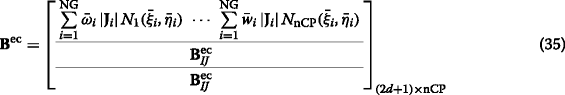$b ecT = 1 0 ⋯ 0 ⏟ d 0 ⋯ 0 ⏟ d$
(36)

where row 1, row 2 to d+1 and row d+2 to 2d+1 in Bec matrix stand for the number of constraints related to an external work rate, the boundary control points and control points adjacent to the boundary, respectively, and $B IJ ec$ is described as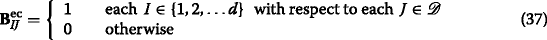where d denotes the number of control points defining the Dirichlet boundary with respect to a set of boundary control points .

Note that first d+1 rows in (35) are indeed used for limit analysis of plates with the only prescribed deflection on Γ1. In addition, when the symmetric boundary conditions are employed, as illustrated in Figure 5, we enforce the constraint of same deflections into the boundary control points and control points adjacent to the symmetric boundary, (i.e. along the symmetry lines, the normal rotation is fixed which can be achieved by enforcing the deflection of two rows of control points that define the tangent of the plate to have the same value ). A matrix storing such constraints will be added to the extensive rows of Bec.Figure 5

It be can observed that the enforcement of essential boundary conditions using the rotation-free approach is simple and efficient in comparison with other numerical methods. For instance, readers can find more details on the advantages of this procedure in ,,,. In addition, IGA based on the Lagrange multiplier, penalty and collocation methods (see ) can be also used to enforce essential boundary conditions for the thin plate.

### 2.3 Solution procedure of the discrete problem

#### 2.3.1 Second-order cone programming

The above limit analysis problem is a non-linear optimization problem with equality constraints. It can be solved using a general non-linear optimization solver such as a sequential quadratic programming (SQP) algorithm (which is a generalization of Newton’s method for unconstrained optimization), a direct iterative algorithm . In particular, the optimization problem can be reduced to the problem of minimizing a sum of norms by Andersen et al.  due to the specific choice of the von Mises criterion and can be reformed as a second-order cone programming (SOCP) problem and solved using an interior point method . The general form of a SOCP problem with Ncs sets of constraints has the following form

$min ∑ i = 1 NG c i t i$
$s. t.∥ H i t+ v i ∥≤ y i T t+ z i fori=1,…, N cs$
(38)

where $t i ∈ℝ,i= 1 , NG ¯$ or $t∈ ℝ NG$ are optimization variables, and the coefficients are $c i ∈ℝ$, $H i ∈ ℝ m dim × NG$, $v i ∈ ℝ m dim$, $y i ∈ ℝ NG$, and $z i ∈ℝ$. For optimization problems in 2D or 3D Euclidean space, mdim=2 or mdim=3, respectively. When mdim=1, the SOCP problem reduces to a linear programming problem.

#### 2.3.2 Solution procedure using second-order cone programming

The limit analysis problem, Equation 31, is a non-linear optimization problem with equality constraints. As stated before, the problem can be reduced to the problem of minimizing a sum of norms following the procedure described by Andersen et al. .

Since Θ is a positive definite matrix, the objective or the internal plastic dissipation function in Equation 31 can be straightforwardly rewritten in a form involving a sum of norms as

$W int ≈ m p ∑ i = 1 NG ω ̄ i J i C T κ ̇ i$
(39)

where · denotes the Euclidean norm appearing in the plastic dissipation function, i.e., v=(vTv)1/2, C is the so-called Cholesky factor of Θ which is given by

$C= 1 3 2 0 0 1 3 0 0 0 1$
(40)

For convenience, a vector of additional variables ρ i is introduced as

$ρ i = ρ 1 ρ 2 ρ 3 T = C T κ ̇ i$
(41)

Hence, Equation 39 becomes

$W int = m p ∑ i = 1 NG ω ̄ i J i ρ i$
(42)

Now the optimization problem (31) becomes a problem of minimizing a sum of norms as

$λ + = min m p ∑ i = 1 NG ω ̄ i J i ρ i s.t ρ i = C T B i w ̇ ∀ i = 1 , NG ¯ B ec w ̇ = b ec$
(43)

In fact, a problem of this sort can be reformed as a SOCP problem by introducing auxiliary variables t1, t2, …, tNG

$λ + = min m p ∑ i = 1 NG ω ̄ i J i t i s.t ρ i ≤ t i ∀ i = 1 , NG ¯ ρ i = C T B i w ̇ ∀ i = 1 , NG ¯ B ec w ̇ = b ec$
(44)

where the first constraint in Equation 44 represents quadratic cones. The total number of variables of the optimization problem is Nvar=NoDofs+4×NG where NoDofs is the total number of the degrees of freedom (DOFs) of the underlying problem. As a result, the optimization problem defined by Equation 44 can be effectively solved by the academic version of the Mosek optimization package .

## 3Results and discussion

In this section, we examine the performance of the present approach through the limit analysis of beams and plates. The computations are performed on a desktop computer with ADM Phenom II X6 (2.8GHz CPU, 16G RAM). For purpose of comparison with other published methods in the literature, we will restrict our interest to simply supported and clamped plates, which are the most frequently found case in practice. As shown in , the standard Gaussian quadrature rule (or nG=(p+1)×(p+1) Gauss points) is used to evaluate the integrals of NURBS elements of p degree. Just a precision, this is valid only for evaluating stiffness matrices and load vector for elastoplastic analyses, but here the dissipation involving a square root cannot be evaluated exactly using Gauss rules. However, we also can utilize this quadrature rule for limit analysis problem without much loss of accuracy of solution. It also is worth mentioning that the computational cost increases significantly when higher-order elements are used. This was pointed out that using the Gauss quadrature rule for NURBS elements is far from optimal. Hence, a simple and efficient quadrature algorithm , for NURBS-based isogeometric analysis will be recommended for our future research. For the limit problem of thin plates, we in this study employ only nG=p×p Gauss pointsa to compute the integral in Equation 27. We also exploit the so-called k-refinement approach, which is a unique characteristic of IGA as a flexible way for refinement and degree elevation for limit analysis problems. Note that with the same number of elements, the total number of DOFs of IGA is less than that of FEM. For all the examples, the von Mises criterion and perfectly rigid plastic material are used.

### 3.1 Beams

We restrict the present formulation to a 1D beam case and verify its performance for the Euler beam of length a and thickness t. Without loss of generality, beams of rectangular cross section (b×t) are considered and subjected to a uniform load and various boundary conditions at the ends, as shown in Figure 6. For computation, a symmetry model is applied to the simply supported and clamped beams whilst the clamped simply supported beam uses a full model.Figure 6

Table 1 shows limit load factors of the beam with various boundary conditions. It can be seen that the numerical results are in very good agreement with analytical solutions. The convergence rates are plotted in Figure 7. They verify the theoretically expected values of 1:1 for the clamped beam and 1:2 for the simply supported beam. Being different from the regularity of the convergence rate in elastic problems , the convergence rates obtained do not change significantly when increasing the degree of basis functions of the approximate solution. This is due to the appearance of plastic hinges in the beam. More importantly, the accuracy of solution is improved with increasing the degree of basis functions.Figure 7

### 3.2 Rectangular plates

We next consider a square plate of length a and thickness t with clamped and simply supported boundary conditions subjected to a uniform load, as shown in Figure 8. Here, we use a symmetric model and a coarsely uniform mesh is given in Figure 8c.Figure 8

Similar to FEM, Gauss quadrature can be utilized for integration on element level, although it was proved that such a Gauss quadrature is far from optimal with NURBS basis functions ,. Herein, discussion focuses on using a right reduction of the number of Gauss points without loss of accuracy of solution. Figure 9 shows that two Gaussian quadrature rules produce the same results while the number of optimization variables using p×p Gauss points reduces very significantly, approximately Nvar/3 less than those using the full integration.Figure 9

For the computational cost, it is estimated based on Nvar versus total optimization steps (Mosek times). Results are plotted in Figure 10. It is seen that the total number of variables increases quickly when increasing the degree of basis functions. Optimization problems solved using the Mosek academic software show very fast convergence in about 13 to 16 step iterations with computing times ranging from 2 to 18 s only.Figure 10

Although the analytical solution is unknown, this benchmark has been investigated by many authors. The earliest numerical performance of the upper and lower bounds was proposed by Hodge and Belytschko . Tables 2 and 3 show the convergence of the present solutions using quadratic and cubic elements versus elemental meshes. The present solutions fall between lower and upper bound published ones.

Table 4 compares the present results with the upper bound solutions ,,,,, the quasi-upper bound  and the (quasi-) lower bound ,. As seen, the limit load factor using the rotation-free IGA approach is more accurate than that of several published solutions. The improved upper bound solution of 45.036 and 44.287 derived with respect to T6b and H3 elements is provided by Bleyer and de Buhan  for the clamped square plate problem. The present results agree well with the most recent upper bounds; one has a value of 44.560 for the cubic B-splines element (between 1 % and 0.6 % with respect to T6b and H3). Figure 11 illustrates several reference upper bound solutions of the clamped square plate and proves the reliability of the present solutions.Figure 11

In addition, Figure 12 depicts the plastic dissipation of simply supported and clamped square plates. It is seen that the rotation-free IGA approach can reproduce properly the plastic dissipation (or yield line mechanism). Even the failure mechanism can be reproduced exactly using a lower number of DOFs.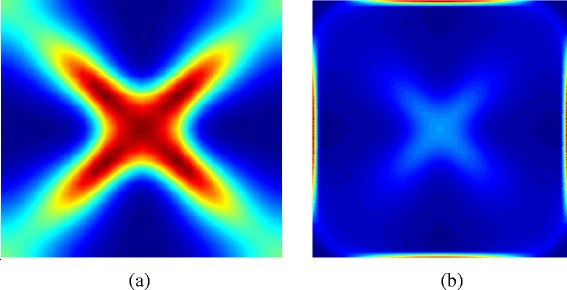Figure 12

Furthermore, to prove the flexibility of the present method, we study its performance using k-refinement (higher order and higher continuity). In this case, two slightly coarse mesh of 4×4 and 8×8 B-spline elements are illustrated. We know that one of the main advantages of IGA is to increase easily the order of basis functions. Especially, k-refinement algorithm in IGA can produce easily C1-continuity between elements while p-refinement (in the p-version FEM) achieves only C0 continuity. The limit load factor of the clamped square plate using the k-refinement algorithm is shown in Figure 13. Numerical results indicate that the rotation-free isogeometric approach with k-refinement improves very well the convergence of solution.Figure 13

Next, let us consider the rectangular plate (length-to-width ratio a/b=2) with various boundary conditions. We compare the present results with other published ones such as the EFG method , the rectangular non-deforming plate element (ACM)  and the C1 natural element method . Table 5 summarizes the limit load multipliers of a rectangular plate with various boundary conditions such as simply supported (SSSS), fully clamped (CCCC), three-clamped and one-short free sides (CCCsF), three-clamped and one-long free sides (CCClF), and two-clamped and two-short free sides (CCsFF). It is again observed that the results obtained are in good accordance with other published ones. Figure 14 shows the plastic dissipation with very good smoothness using the cubic B-spline basis functions.Figure 14

### 3.3 Rhombic plate

A rhombic plate with varying skewness angles α as shown in Figure 15 is subjected to a uniform load. For comparison, the rhombic plate is modeled by 20×20 B-spline elements, as shown in Figure 16. Simply supported and fully clamped boundary conditions are considered. In the numerical calculation, the radius of the circular in Figure 16 is chosen as R=0.5 and the plate thickness is fixed at t=0.02. The results of the collapse limit factor with varying skewness angle are listed in Table 6. The obtained result is compared with the analytical solution given by Mansfield , the Mindlin plate finite element (N4$B ̄ 0$) proposed by Capsoni and Silva  and C1 natural element method (C1-NEM) based on Kirchhoff plate model by Zhou et al. . For this problem, an analytical approach into lower bound (LB) and upper bound solutions using the square yield criterion were proposed by Mansfield . Note that an upper bound (UB) solution using the square yield criterion can be obtained by multiplying the lower bound value with a factor of $2/ 3$. Figure 17 depicts the collapse limit factor with respect to various skewness angles. It is observed that the result of the quadratic element matches well with that of the N4$B ̄ 0$ element. The cubic element produces higher accuracy solutions than the N4$B ̄ 0$ element and is competitive to the C1-NEM. When increasing skewness angle, the limit load factor value decreases. Figure 18 shows the plastic dissipation energy of 60°-rhombic plates using the cubic element. It is clear that the present method is reliable for predicting collapse mechanism compared to other published ones ,.Figure 15Figure 16Figure 17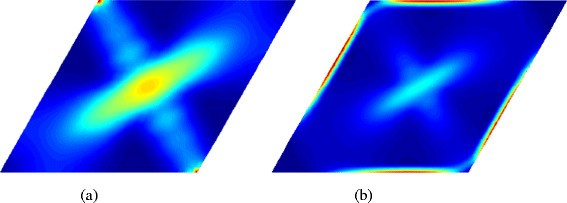Figure 18

### 3.4 L-shaped plate

We consider an L-shaped plate subjected to a uniform load. The plate geometry is shown in Figure 19. Control net, coarse mesh and fine mesh are detailed in Figure 20. Knot vectors and control data are given in the Appendix (‘L-shaped plate’). This problem involves a singular behaviour at the obtuse vertices. Simply supported and fully clamped boundary conditions are used. The plate is modeled by 50×25 B-spline elements of 1,512 nodes (Nvar=21,512 for p=2, and Nvar=46513 for p=3). The obtained results are compared with those of the quasi-upper approach based on the element-free method  and the dual FEM approach  based on the conforming HCT and enhanced equilibrium Morley (EM) element using the finest mesh of 6,426 nodes (or Nvar=105,264 for HCT). Table 7 summarizes the collapse limit load factor. The present elements provide strict upper bound solutions and produce load factor values lower than EFG and HCT elements. Note that the HCT element has 3 Dofs per node resulting in a larger number of DOFs than the present elements. Also, for comparison, the EFG requires 3,816 nodes (or 3,816 NoDofs) while the cubic element has only 1,024 nodes.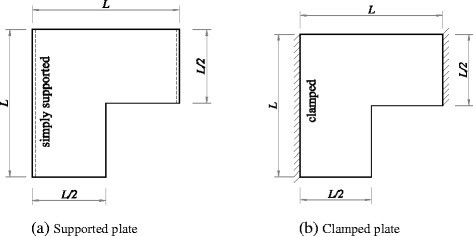Figure 19Figure 20

The accuracy of the present method in comparison with HCT element is also shown in Figure 21. It is clear that the performance of the present elements is better than that of the HCT.Figure 21

### 3.5 Circular plate

#### 3.5.1 Circular plate subjected to uniform transverse loading

In this example, we consider a fully clamped circular plate under uniform transverse loading as illustrated in Figure 22. The circular plate has a radius to thickness ratio of 100 (R/t=100). A rational quadratic basis is enough to model exactly the circular geometry. Knot vectors and control data of the circular plate are given in the Appendix (‘Circular plate’). Coarse mesh and control net of the plate with respect to quadratic, cubic, quartic and fifteenth elements are illustrated in Figure 23. To investigate the convergence of the limit load factor, different meshes of 4×4,12×12,20×20 and 28×28 NURBS elements are displayed in Figure 24. The results of the limit load factor are provided in Figure 25. The obtained solution is compared with that of the analytical approach  and the numerical formulation presented in Capsoni and Silva . It can be seen that the present results converge well to the analytical value and are much better than those of the N4$B ̄ 0$ element.Figure 22Figure 23Figure 24Figure 25

The k-refinement algorithm is now used to calculate the limit load factor of the clamped circular plate. Figure 26 shows the limit load factor of various degrees of NURBS functions (from 5 to 15) based on a mesh of 6×6 NURBS elements. The obtained result converges well to the best reference value given in the literature.Figure 26

#### 3.5.2 Circular plate subjected to non-uniform transverse loading

Finally, we study a clamped circular plate subjected to non-uniform (linear and parabolic) load as shown in Figure 27. The parabolic load can be written as follows :

$f(r)= a 1 + a 2 r+ a 3 r 2$
(45)Figure 27

where a1, a2 and a3 are predefined constants. In the numerical calculation, the constants a1, a2 and a3 are chosen in such a way that a1 is fixed at value of 3 (a1=3) and a2,a3 vary. The geometry and material parameters are as given in the previous case. For illustration, we use a slightly fine mesh of 17×17 NURBS elements for quadratic and cubic elements.

For a varying load case, the analytically upper bound value based on the square yield criterion is $λ cr =12 3 m p / 2 a 1 + a 2 R R 2$. Table 8 compares the present solutions and the analytical values using both the Tresca and square yield criteria reported by Ghorashi . As expected, the present results almost vary between the upper bound solutions using the Tresca and square yield criteria.

For a parabolic load case, the constants are chosen such as a1=3, a2=2 and various a3 values. The limit load factor is given in Table 9. Again, the present solutions are bounded by the upper bound values reported in .

## 4Conclusions

We have for the first time presented a rotation-free isogeometric finite element approach for upper bound limit analysis of thin plate structures. The method was derived from the kinematic theorem and isogeometric finite elements. The underlying optimization formulation of limit analysis was transformed into the form of a second-order cone programming, and it was then solved by highly efficient interior-point solvers. The performance of the method is validated through benchmark problems of plastic thin plates. Through the examples tested, some concluding remarks can be given as follows:

Only deflection degrees of freedom were needed in the optimization problem. Thus, the method requires less variables than N4$B ̄ 0$ element (or C0-FEM), HCT element (or C1-FEM) and C1 natural element (C1-NEM). As a result, it is promising to provide an effective way to solve large-scale plate problems.

The essential boundary conditions are easily imposed in the context of the rotation-free isogeometric approach.

Beyond h-and p-refinement schemes currently available in the traditional FEM, the present approach was found to be more efficient with k-refinement type for limit analysis of thin plates.

Numerical results showed that the present method provides upper bound estimates of collapse limit loads, that proves the stability of the method. Also, the proposed method exhibited very good agreement with several published results in the literature for different benchmark problems. It seems, in particular, very efficient for the L-shaped plate problem which presents a singularity near the corner.

In present work, only benchmark problems were used to show the performance of the proposed formulation. However, we believe that the methodology is generalizable for large-scale plate problems in practice. Although the present method achieved high reliability, its computational cost is still significant due to an excessive overhead of control points for very uniformly refined meshes. It would therefore be interesting to associate the present method with adaptive local refinement procedures ,. This is a work in progress and our findings will be devoted in a forthcoming paper.

## 5Endnotes

a This helps to reduce the size of the optimization problem without loss of accuracy of solution as it will be shown later.

## 6Appendix

### 6.1 Knot vectors and control points for NURBS objects

#### 6.1.1 Circular plate

A circular plate is shown in Figure 22. A rational quadratic basis is used to exactly describe the geometry of the circular plate. Knot vectors of the coarsest mesh with one element is defined as follows: Ξ={0,0,0,1,1,1}; . Data of the circular plate are given in Table 10.

#### 6.1.2 L-shaped plate

An L-shaped plate is illustrated in Figure 20. As stated previously, a rational quadratic basis is used to describe an L-shaped plate. Knot vectors of the coarsest mesh with two elements are defined as follows: Ξ={0,0,0,0.5,1,1,1}; . Control points of the L-shaped plate are given in Table 11. Note that all control points have unit weights.

## References

1. 1.

Hodge PG, Belytschko T: Numerical methods for the limit analysis of plates. J Appl Mech 1968,35(4):795–802. 10.1115/1.3601308

2. 2.

Capsoni A, Corradi L: Limit analysis of plates - a finite element formulation. Struct Eng Mech 1999, 8: 325–341. 10.12989/sem.1999.8.4.325

3. 3.

Le CV, Nguyen-Xuan H, Nguyen-Dang H: Dual limit analysis of plate bending. In Collection of papers (modeling in mechanical and civil engineering) from Prof. Nguyen-Dang Hung’s former students.. Vietnam National University Ho Chi Minh City Publishing House, Ho Chi Minh; 2006:476–494.

4. 4.

Le CV, Nguyen-Xuan H, Nguyen-Dang H: Upper and lower bound limit analysis of plates using FEM and second-order cone programming. Comput Struct 2010, 88: 65–73. 10.1016/j.compstruc.2009.08.011

5. 5.

Tran TN: A dual algorithm for shakedown analysis of plate bending. Int J Numer Meth Eng 2011,86(7):862–875. 10.1002/nme.3081

6. 6.

Bleyer J, Buhan P: On the performance of non-conforming finite elements for the upper bound limit analysis of plates. Int J Numer Meth Eng 2013, 94: 308–330. 10.1002/nme.4460

7. 7.

Le CV, Gilbert M, Askes H: Limit analysis of plates using the EFG method and second-order cone programming. Int J Numer Meth Eng 2009,78(13):1532–1552. 10.1002/nme.2535

8. 8.

Le CV, Askes H, Gilbert M: Adaptive element-free Galerkin method applied to the limit analysis of plates. Comput Meth Appl Mech Eng 2010, 199: 2487–2496. 10.1016/j.cma.2010.04.004

9. 9.

Zhou S, Liu Y, Chen S: Upper bound limit analysis of plates utilizing the C1 natural element method. Comput Mech 2012, 50: 543–561. 10.1007/s00466-012-0688-8

10. 10.

Andersen KD, Christiansen E, Overton ML: An efficient primal-dual interior-point method for minimizing a sum of Euclidean norms. SIAM J Sci Comput 2001, 22: 243–262. 10.1137/S1064827598343954

11. 11.

Andersen ED, Roos C, Terlaky T: On implementing a primal-dual interior-point method for conic quadratic programming. Math Program 2003, 95: 249–277. 10.1007/s10107-002-0349-3

12. 12.

Mosek (2009) The MOSEK optimization toolbox for MATLAB manual. Mosek ApS, version 5.0 edition.., [http://www.mosek.com]

13. 13.

Ciria H, Peraire J, Bonet J: Mesh adaptive computation of upper and lower bounds in limit analysis. Int J Numer Meth Eng 2008, 75: 899–944. 10.1002/nme.2275

14. 14.

Makrodimopoulos A, Martin CM: Upper bound limit analysis using simplex strain elements and second-order cone programming. Int J Numer Anal Meth Geomech 2006, 31: 835–865. 10.1002/nag.567

15. 15.

Munoz JJ, Bonet J, Huerta A, Peraire J: Upper and lower bounds in limit analysis: adaptive meshing strategies and discontinuous loading. Int J Numer Meth Eng 2009, 77: 471–501. 10.1002/nme.2421

16. 16.

Le CV, Gilbert M, Askes H: Limit analysis of plates and slabs using a meshless equilibrium formulation. Int J Numer Meth Eng 2010, 83: 1739–1758. 10.1002/nme.2887

17. 17.

Hughes TJR, Cottrell JA, Bazilevs Y: Isogeometric analysis: CAD, finite elements, NURBS, exact geometry and mesh refinement. Comput Methods Appl Mech Eng 2005,194(39–41):4135–4195. 10.1016/j.cma.2004.10.008

18. 18.

Cottrell J, Hughes TJR, Reali A: Studies of refinement and continuity in isogeometric analysis. Comput Methods Appl Mech Eng 2007, 196: 4160–4183. 10.1016/j.cma.2007.04.007

19. 19.

Cottrell JA, Reali A, Bazilevs Y, Hughes TJR: Isogeometric analysis of structural vibrations. Comput Methods Appl Mech Eng 2006,195(41–43):5257–5296. 10.1016/j.cma.2005.09.027

20. 20.

Elguedj T, Bazilevs Y, Calo V, Hughes T: B and F projection methods for nearly incompressible linear and non-linear elasticity and plasticity using higher-order NURBS elements. Comput Methods Appl Mech Eng 2008, 197: 2732–2762. 10.1016/j.cma.2008.01.012

21. 21.

Wall WA, Frenzel MA, Cyron C: Isogeometric structural shape optimization. Comput Methods Appl Mech Eng 2008,197(33–40):2976–2988. 10.1016/j.cma.2008.01.025

22. 22.

Kiendl J, Bletzinger KU, Linhard J, Wüchner R: Isogeometric shell analysis with Kirchhoff–Love elements. Comput Methods Appl Mech Eng 2009,198(49–52):3902–3914. 10.1016/j.cma.2009.08.013

23. 23.

Kiendl J, Bazilevs Y, Hsu MC, Wüchner R, Bletzinger KU: The bending strip method for isogeometric analysis of Kirchhoff–Love shell structures comprised of multiple patches. Comput Methods Appl Mech Eng 2010,199(37–40):2403–2416. 10.1016/j.cma.2010.03.029

24. 24.

Benson DJ, Bazilevs Y, Hsu MC, Hughes TJR: Isogeometric shell analysis: the Reissner–Mindlin shell. Comput Methods Appl Mech Eng 2010,199(5–8):276–289. 10.1016/j.cma.2009.05.011

25. 25.

Nguyen-Thanh N, Kiendl J, Nguyen-Xuan H, Wuchner R, Bletzinger KU, Bazilevs Y, Rabczuk T: Rotation free isogeometric thin shell analysis using PHT-splines. Comput Methods Appl Mech Eng 2011,200(47–48):3410–3424. 10.1016/j.cma.2011.08.014

26. 26.

Benson DJ, Bazilevs Y, Hsu MC, Hughes TJR: A large deformation, rotation-free, isogeometric shell. Comput Methods Appl Mech Eng 2011,200(13–16):1367–1378. 10.1016/j.cma.2010.12.003

27. 27.

Simpson RN, Bordas SPA, Trevelyan J, Rabczuk T: A two-dimensional isogeometric boundary element method for elastostatic analysis. Comput Methods Appl Mech Eng 2012, 209–212: 87–100. 10.1016/j.cma.2011.08.008

28. 28.

Reddy JN: Theory and analysis of elastic plates and shells. CRC Press, Taylor and Francis Group, Boca Raton; 2007.

29. 29.

Oñate E, Zarate F: Rotation-free triangular plate and shell elements. Int J Numer Meth Eng 2000, 47: 557–603. 10.1002/(SICI)1097-0207(20000110/30)47:1/3<557::AID-NME784>3.0.CO;2-9

30. 30.

Oñate E, Flores FG: Advances in the formulation of the rotation-free basic shell triangle. Comput Methods Appl Mech Eng 2005, 194: 2406–2443. 10.1016/j.cma.2004.07.039

31. 31.

Flores FG, Estrada CF: A rotation-free thin shell quadrilateral. Comput Methods Appl Mech Eng 2007, 196: 2631–2646. 10.1016/j.cma.2007.01.008

32. 32.

Flores FG, Oñate E: Wrinkling and folding analysis of elastic membranes using an enhanced rotation-free thin shell triangular element. Comput Methods Appl Mech Eng 2007, 196: 2631–2646. 10.1016/j.cma.2007.01.008

33. 33.

Piegl LA, Tiller W: The NURBS book. Springer Verlag, Heidelberg; 1997.

34. 34.

Cottrell JA, Reali A, Bazilevs Y, Hughes TJR: Isogeometric analysis of structural vibrations. Comput Methods Appl Mech Eng 2006, 195: 5257–5297. 10.1016/j.cma.2005.09.027

35. 35.

Cottrell JA, Hughes TJR, Bazilevs Y: Isogeometric analysis toward integration of CAD and FEA. Wiley, New York; 2009.

36. 36.

Christiansen E: Limit analysis of collapse states. In Handbook of numerical analysis, vol IV, chapter II. North-Holland, Amsterdam; 1996:193–312.

37. 37.

Auricchio F, Beirao da Veiga L, Buffa A, Lovadina C, Reali A, Sangalli G: A fully locking-free isogeometric approach for plane linear elasticity problems: a stream function formulation. Comput Methods Appl Mech Eng 2007, 197: 160–172. 10.1016/j.cma.2007.07.005

38. 38.

Capsoni A, Corradi L: A finite element formulation of the rigid-plastic limit analysis problem. Int J Numer Meth Eng 1997, 40: 2063–2086. 10.1002/(SICI)1097-0207(19970615)40:11<2063::AID-NME159>3.0.CO;2-#

39. 39.

Andersen KD, Christiansen E, Overton ML: Computing limit loads by minimizing a sum of norms. SIAM J Sci Comput 1998, 19: 1046–1062. 10.1137/S1064827594275303

40. 40.

Hughes TJR, Reali A, Sangalli G: Efficient quadrature for NURBS-based isogeometric analysis. Comput Methods Appl Mech Eng 2010, 199: 301–313. 10.1016/j.cma.2008.12.004

41. 41.

Auricchio F, Calabroo F, Hughes TJR, Reali A, Sangalli G: A simple algorithm for obtaining nearly optimal quadrature rules for NURBS-based isogeometric analysis. Comput Methods Appl Mech Eng 2012, 249–252: 15–27. 10.1016/j.cma.2012.04.014

42. 42.

Melosh RJ: Basis for derivation of matrices for the direct stiffness method. J Am Inst Aeronaut Astronautics 1963,1(7):1631–1637. 10.2514/3.1869

43. 43.

Ghorashi M: Limit analysis of circular plates subjected to arbitrary rotational symmetric loadings. Int J Mech Sci 1994,36(2):87–94. 10.1016/0020-7403(94)90077-9

44. 44.

Capsoni A, Silva MV: A finite element formulation of Mindlin plates for limit analysis. Int J Numerical Methods Biomed Eng 2011,27(1):143–156. 10.1002/cnm.1300

45. 45.

Cinquini C, Zanon P: Limit analysis of circular and annular plates. Ingenier - Archiv 1985, 55: 157–175. 10.1007/BF00536411

46. 46.

Bazilevs Y, Calo VM, Cottrell JA, Evans JA, Hughes TJR, Lipton S, Scott MA, Sederberg TW: Isogeometric analysis using T-splines. Comput Methods Appl Mech Eng 2010,199(5–8):229–263. 10.1016/j.cma.2009.02.036

Download references

## Acknowledgments

This research is funded by Vietnam National Foundation for Science and Technology Development (NAFOSTED) under grant number 107.02-2014.24. The support is gratefully acknowledged.

## Author information

Authors

### Corresponding author

Correspondence to Hung Nguyen-Xuan.

## Additional information

### Competing interests

The authors declare that they have no competing interests.

### Authors’ contributions

HXN provided an original idea of further developing the rotation-free isogeometric approach in upper bound limit analysis of plates, developed the code, and revised the manuscript. CHT carried out the numerical examples and drafted the manuscript. JB revised the manuscript. PVN provided the source code of isogeometric analysis and revised the manuscript. All authors read and approved the final manuscript.

## Authors’ original submitted files for images

Below are the links to the authors’ original submitted files for images.

## Rights and permissions

Open Access This article is distributed under the terms of the Creative Commons Attribution 4.0 International License (https://creativecommons.org/licenses/by/4.0), which permits use, duplication, adaptation, distribution, and reproduction in any medium or format, as long as you give appropriate credit to the original author(s) and the source, provide a link to the Creative Commons license, and indicate if changes were made.

Reprints and Permissions